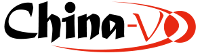# VizieRI/337/gaia Gaia DR1 (Gaia Collaboration, 2016) ReadMe+ftpSample of GaiaSource data (Download Gaia Sources as vo table, fits or csv here) (original column names in green) (1142679769 rows) -c=289.2071800578 +47.8676940514, eq=ICRS, rs=2
 Column Value Explain   (UCD) _r 0.000 arcsec Distance from center (289.2071800578+47.8676940514)[ICRS], at Epoch=J2015.0 (POS_ANG_DIST_GENERAL) _RAJ2000 19 16 49.723213872 "h:m:s" Right ascension (FK5, Equinox=J2000.0) at Epoch=J2000, proper motions taken into account (POS_EQ_RA) _DEJ2000 +47 52 03.69858504 "d:m:s" Declination (FK5, Equinox=J2000.0) at Epoch=J2000, proper motions taken into account (POS_EQ_DEC) _RAB1950 19 15 25.645644889 "h:m:s" Right ascension (FK4, Equinox=B1950.0) at Epoch=J1950, proper motions taken into account (POS_EQ_RA) _DEB1950 +47 46 36.87756865 "d:m:s" Declination (FK4, Equinox=B1950.0) at Epoch=J1950, proper motions taken into account (POS_EQ_DEC) _Glon 079.073047738964 deg Galactic longitude at Epoch=J2015, proper motions taken into account (POS_GAL_LON) _Glat +15.792025020279 deg Galactic latitude at Epoch=J2015, proper motions taken into account (POS_GAL_LAT) ra 289.2071800578 deg (i) Right ascension (ICRS) at epoch 2015.0 (ra) (POS_EQ_RA_MAIN) ra_error 0.309 mas Standard error of right ascension (ra_error) (ERROR) dec +47.8676940514 deg (i) Declination (ICRS) at epoch 2015.0 (dec) (POS_EQ_DEC_MAIN) dec_error 0.510 mas Standard error of declination (dec_error) (ERROR) solution_id 1635378410781933568 Solution ID (solution_id) (Note G1)    (ID_NUMBER) source_id 2127941375008154880 (i) Source ID (source_id) (Note G2)    (ID_MAIN) random_index 144449703 Random index used to select subsets (random_index) (Note G3)    (CODE_MISC) ref_epoch 2015.0 yr [2015.0] Reference epoch in Julian years (ref_epoch) (Note G4)    (TIME_EPOCH) parallax mas (i) Absolute barycentric stellar parallax of the source at the reference epoch Epoch (parallax) (POS_PARLX_TRIG) parallax_error mas Standard error of parallax (parallax_error) (ERROR) pmRA mas/yr Proper motion in right ascension direction (pmRA) (Note G5)    (POS_EQ_PMRA) pmra_error mas/yr Standard error of proper motion in right ascension direction (pmra_error) (ERROR) pmdec mas/yr Proper motion in declination direction (pmdec) (Note G6)    (POS_EQ_PMDEC) pmdec_error mas/yr Standard error of proper motion in declination direction (pmdec_error) (ERROR) ra_dec_corr 0.738 Correlation between right ascension and declination (ra_dec_corr) (STAT_CORRELATION) ra_parallax_corr Correlation between right ascension and parallax (ra_parallax_corr) (STAT_CORRELATION) ra_pmra_corr Correlation between right ascension and proper motion in right ascension (ra_pmra_corr) (STAT_CORRELATION) ra_pmdec_corr Correlation between right ascension and proper motion in declination (ra_pmdec_corr) (STAT_CORRELATION) dec_parallax_corr Correlation between declination and parallax (dec_parallax_corr) (STAT_CORRELATION) dec_pmra_corr Correlation between declination and proper motion in right ascension (dec_pmra_corr) (STAT_CORRELATION) dec_pmdec_corr Correlation between declination and proper motion in declination (dec_pmdec_corr) (STAT_CORRELATION) parallax_pmra_corr Correlation between parallax and proper motion in right ascension (parallax_pmra_corr) (STAT_CORRELATION) parallax_pmdec_corr Correlation between parallax and proper motion in declination (parallax_pmdec_corr) (STAT_CORRELATION) pmra_pmdec_corr Correlation between proper motion in right ascension and proper motion in declination (pmra_pmdec_corr) (STAT_CORRELATION) astrometric_n_obs_al 94 Total number of observations AL (astrometric_n_obs_al) (Note G7)    (NUMBER) astrometric_n_obs_ac 0 Total number of observations AC (astrometric_n_obs_ac) (Note G8)    (NUMBER) astrometric_n_good_obs_al 94 Number of good observations AL (astrometric_n_good_obs_al) (Note G9)    (NUMBER) astrometric_n_good_obs_ac 0 Number of good observations AC (astrometric_n_good_obs_ac) (Note G9)    (NUMBER) astrometric_n_bad_obs_al 0 Number of bad observations AL (astrometric_n_bad_obs_al) (Note G10)    (NUMBER) astrometric_n_bad_obs_ac 0 Number of bad observations AC (astrometric_n_bad_obs_ac) (Note G10)    (NUMBER) astrometric_delta_q 0.00 Hipparcos/Gaia data discrepancy (Hipparcos subset of TGAS only) (astrometric_delta_q) (Note G11)    (STAT_PARAM) astrometric_excess_noise 0.25 mas Excess noise of the source (astrometric_excess_noise) (Note G12)    (STAT_PARAM) astrometric_excess_noise_sig 2.42e-01 [] Significance of excess noise (astrometric_excess_noise_sig) (G13) (Note G13)    (STAT_PARAM) astrometric_primary_flag 0 [0/1] Primary or secondary (astrometric_primary_flag) (Note G14)    (CODE_MISC) astrometric_relegation_factor 1.02 Relegation factor (astrometric_relegation_factor) (STAT_PARAM) astrometric_weight_al 0.516 mas-2 Mean astrometric weight of the source in the AL direction (astrometric_weight_al) (WEIGHT) astrometric_weight_ac mas-2 Mean astrometric weight of the source in the AC direction (astrometric_weight_ac) (WEIGHT) astrometric_priors_used 2 [0/6] Type of prior used in in the astrometric solution (astrometric_priors_used) (Note G15)    (CODE_MISC) matched_observations 16 Amount of observations matched to this source (matched_observations) (Note G16)    (NUMBER) duplicated_source 0 [0/1] Source with duplicate sources (duplicated_source) (Note G17)    (CODE_MISC) scan_direction_strength_k1 0.100 [0/1] Degree of concentration of scan directions across the source (scan_direction_strength_k1) (Note G18)    (CODE_MISC) scan_direction_strength_k2 0.323 [0/1] Degree of concentration of scan directions across the source (scan_direction_strength_k2) (Note G18)    (CODE_MISC) scan_direction_strength_k3 0.413 [0/1] Degree of concentration of scan directions across the source (scan_direction_strength_k3) (Note G18)    (CODE_MISC) scan_direction_strength_k4 0.434 [0/1] Degree of concentration of scan directions across the source (scan_direction_strength_k4) (Note G18)    (CODE_MISC) scan_direction_mean_k1 -31.95699100 deg Mean position angle of scan directions across the source (scan_direction_mean_k1) (Note G19)    (CODE_MISC) scan_direction_mean_k2 -68.00449000 deg Mean position angle of scan directions across the source (scan_direction_mean_k2) (Note G19)    (CODE_MISC) scan_direction_mean_k3 43.58427400 deg Mean position angle of scan directions across the source (scan_direction_mean_k3) (Note G19)    (CODE_MISC) scan_direction_mean_k4 7.02747630 deg Mean position angle of scan directions across the source (scan_direction_mean_k4) (Note G19)    (CODE_MISC) phot_g_n_obs 99 Number of observations contributing to G photometry (phot_g_n_obs) (NUMBER) phot_g_mean_flux 8.1943e+02 e-/s (i) G-band mean flux (phot_g_mean_flux) (PHOT_FLUX_R) phot_g_mean_flux_error 7.4347e+00 e-/s (i) Error on G-band mean flux (phot_g_mean_flux_error) (ERROR) phot_g_mean_mag 18.241 mag (i) G-band mean magnitude (phot_g_mean_mag) (Note G20)    (PHOT_MAG_R) phot_variable_flag NOT_AVAILABLE Photometric variability flag (phot_variable_flag) (Note G21)    (CODE_VARIAB) l 79.0730501190 deg (i) Galactic longitude at epoch 2015.0 (l) (POS_GAL_LON) b 15.7920235062 deg (i) Galactic latitude at epoch 2015.0 (b) (POS_GAL_LAT) ecl_lon 307.6334370801 deg Ecliptic longitude at epoch 2015.0 (ecl_lon) (POS_EC_LON) ecl_lat 68.8113308418 deg Ecliptic latitude at epoch 2015.0 (ecl_lat) (POS_EC_LAT)

The following problems were encountered in this VizieR run:
```****catClient fails: (XmatchCatError) [Errno 111] Connection refused
```
If you can't find a solution, report to CDS and insert into your message the following details:

```.errorfile=/tmp/VR03629.err (2022-08-19T04:21:19)
-5
-ref=VIZ62c1aa8c56fd
-ref=VIZ62c1aa8c56fd